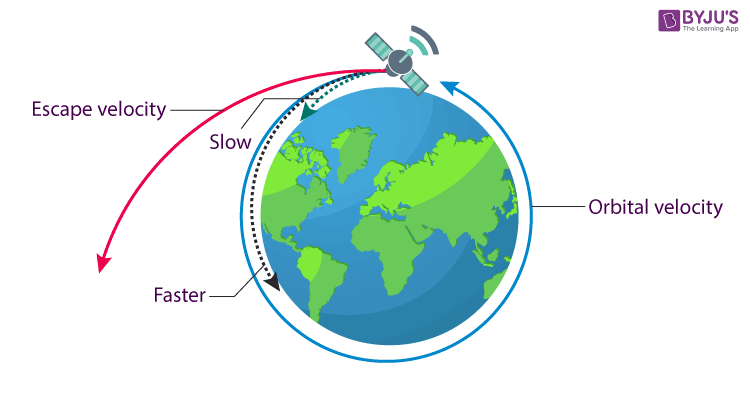# Gravity IIT JEE Study Material

The natural phenomenon by which all the objects with mass are brought towards one another, including objects like atoms, photons, stars, planets, etc is called gravity or acceleration due to gravity. Its S.I unit is m s -2.

## Universal Law of Gravitation

Somewhere in between, a great man called Newton watched an apple fall from a tree and rather than picking it up and eating it as most of us would have, he wondered what invisible forces drive the apple to fall down.

Science has described gravity as a certain attractive force exerted by all the objects in the universe. According to the universal law of gravitation, every single object in the universe attracts every other object with a force directed along the line of centres for the two objects that is proportional to the product of their masses and inversely proportional to the square of the separation between the two objects.

F ∝ m1m2/d2

F = G (m1m2/d2 )

Here, m1 and m2 are the masses of the two particles

d is the distance between the two masses

G is the gravitational constant = 6.673 x 10-11 Nm2kg-2

The law of gravitation led to the explanation of Kepler’s laws and terrestrial gravitation.

Gravity exists everywhere. But the moon just does not have a strong gravity as the earth. One might wonder if this force of gravity is all prevalent, then why don’t the chairs and tables jump at each other?

This is because the force of gravity of the earth is much stronger than the chair and table’s force of gravity. It also depends on how far away you are from this force of gravity. The farther away you go from the earth, the less the force of gravity affects you.

## Gravitational Potential Energy

The energy possessed by an object because of its position in the gravitational field is called the gravitational potential energy. The equation of gravitational potential energy can be got from the law of gravity and it is equal to the amount of work done against gravity to bring the mass to the given point in space. The gravitational force is inversely proportional to the square of the distance, therefore the force will be zero for a large distance. So, the gravitational potential energy will be negative near a planet since gravity does positive work as the mass approaches.  The expression for gravitational potential energy associated with two particles separated by a distance r is given as

U = – Gm1m2/r

This expression is most useful to calculate the escape velocity.

## Gravitational Potential Energy of a System:

Change in gravitational potential energy U (r) of two point masses m1and m2as their separation is changed from r1to r2is given by,

$\mathbf{U\left ( r_{2} \right )\;-\;U\left ( r_{1} \right )\;=\;\frac{G\;m_{1}\;m_{2}}{r_{1}\;-\;r_{2}}}$

At infinite separation the gravitational potential energy (Ur) is assumed to be 0,

i.e. $\mathbf{U\left ( r \right )\;=\;-\;\frac{G\;m_{1}\;m_{2}}{r}}$

## Gravitational Potential (V)

The gravitational potential at any point is the amount of work done per unit mass that is required to move an object to that point. The gravitational potential at any point is the gravitational potential energy per unit mass.

V = U/m

U is the gravitational potential energy

m is the mass of the object

The mathematical expression for the potential is given by

$V(x)=\frac{W}{m}=\frac{1}{m}\int_{\infty }^{x}F.dx$ $=\frac{1}{m}\int_{\infty }^{x}\frac{GmM}{x^{2}}.dx = -\frac{GM}{x}$

## Gravitational Potential (V) due to Uniform Solid Sphere:

A. At distance r from the centre (Outside the sphere)

$\mathbf{V\;=\;-\;\frac{GM}{r}}$

B.At distance r from the centre (Inside the sphere)

$\mathbf{V\;=\;-\;\frac{GM}{R^{3}}\;\left [ \frac{R^{2}}{2} \;-\;\frac{r^{2}}{6} \right ]}$

## Escape Velocity:

The escape velocity is the minimum velocity given to an object to make it free from the gravitational field. Let vbe the escape velocity of the body and m be the mass of the object to be projected then,

$\mathbf{v_{e}\;=\;\sqrt{\frac{2\;G\; M}{R}}}$Don’t you hate falling objects, especially when they land on you? God forbid a crow flies over your head and suddenly decides to take a dump. Or worse yet, a much heavier object falls over you, well then you’ll meet your maker soon enough. But fear not, if nothing else I shall tell you where to point your finger or whom or rather what to blame, gravity. We, as a race have come far, from rubbing two stones together, watching the sparks and the resultant fire with awe and curiosity to building robots.

Humans have accomplished something extraordinary. We were never meant to fly. But we overcame gravity, built flights and aircraft to soar the skies. Now every time you catch an apple that’s about to fall, you know that you’ve defied gravity.